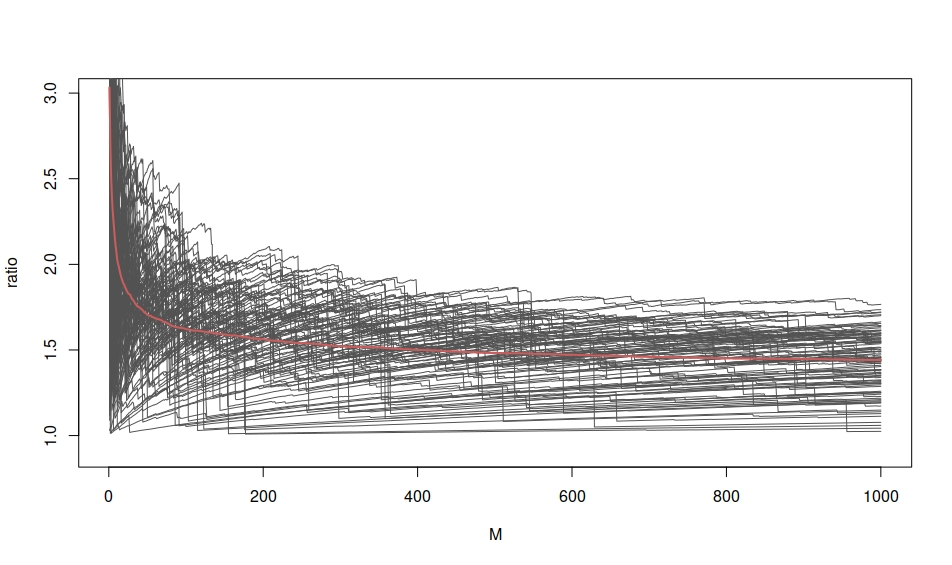Want to share your content on R-bloggers? click here if you have a blog, or here if you don't.$\mathbb E^{U,V}\left[\sum_{i=1}^M U_i\Big/\sum_{i=1}^M U_i/V_i \right]\quad U_i,V_i\sim\mathcal U(0,1)$

when $M$ increases. Actually, this expectation is asymptotically equivalent to$\mathbb E^{V}\left[M\big/\sum_{i=1}^M 2U_i/V_i \right]\quad U_i,V_i\sim\mathcal U(0,1)$

or again$\mathbb E^{V}\left[1\big/(1+2\overline R_{M/2})\right]$

where the average is made of Pareto (1,1), since one can invoke Slutsky many times. (And the above comparison of the integrated rv’s does not show a major difference.) Comparing several Monte Carlo sequences shows a lot of variability, though, which is not surprising given the lack of expectation of the Pareto (1,1) distribution. But over the time I spent on that puzzle last week end, I could not figure the limiting value, despite uncovering the asymptotic behaviour of the average.